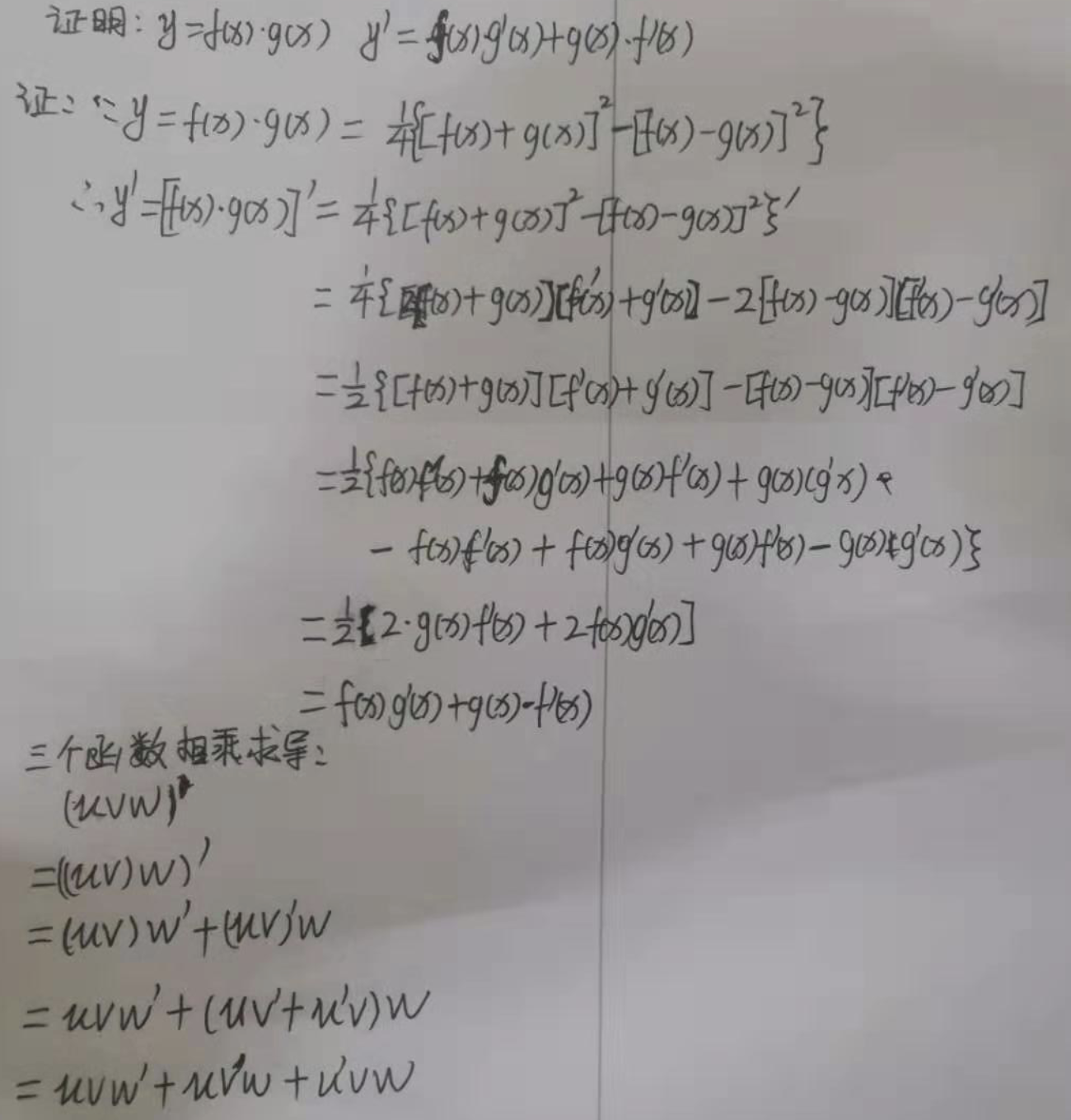• ## 矩阵 相乘求导

万次阅读 2017-11-18 21:27:42
矩阵求导 属于 矩阵计算，应该查找 Matrix Calculus 的文献： http://www.psi.toronto.edu/matrix/intro.html#Intro http://www.psi.toronto.edu/matrix/calculus.html http://www.stanford.edu/~dattorro/...

矩阵求导 属于 矩阵计算，应该查找 Matrix Calculus 的文献：

http://www.psi.toronto.edu/matrix/intro.html#Intro

http://www.psi.toronto.edu/matrix/calculus.html

http://www.stanford.edu/~dattorro/matrixcalc.pdf

http://www4.ncsu.edu/~pfackler/MatCalc.pdf

http://center.uvt.nl/staff/magnus/wip12.pdf

在网上看到有人贴了如下求导公式：

Y = A * X --> DY/DX = A'
Y = X * A --> DY/DX = A
Y = A' * X * B --> DY/DX = A * B'
Y = A' * X' * B --> DY/DX = B * A'

于是把以前学过的矩阵求导部分整理一下：

1. 矩阵Y对标量x求导：

相当于每个元素求导数后转置一下，注意M×N矩阵求导后变成N×M了

Y = [y(ij)] --> dY/dx = [dy(ji)/dx]

2. 标量y对列向量X求导：

注意与上面不同，这次括号内是求偏导，不转置，对N×1向量求导后还是N×1向量

y = f(x1,x2,..,xn) --> dy/dX = (Dy/Dx1,Dy/Dx2,..,Dy/Dxn)'

3. 行向量Y'对列向量X求导：

注意1×M向量对N×1向量求导后是N×M矩阵。

将Y的每一列对X求偏导，将各列构成一个矩阵。

重要结论：

dX'/dX = I

d(AX)'/dX = A'

4. 列向量Y对行向量X’求导：

转化为行向量Y’对列向量X的导数，然后转置。

注意M×1向量对1×N向量求导结果为M×N矩阵。

dY/dX' = (dY'/dX)'

5. 向量积对列向量X求导运算法则：

注意与标量求导有点不同。

d(UV')/dX = (dU/dX)V' + U(dV'/dX)

d(U'V)/dX = (dU'/dX)V + (dV'/dX)U'

重要结论：

d(X'A)/dX = (dX'/dX)A + (dA/dX)X' = IA + 0X' = A

d(AX)/dX' = (d(X'A')/dX)' = (A')' = A

d(X'AX)/dX = (dX'/dX)AX + (d(AX)'/dX)X = AX + A'X

6. 矩阵Y对列向量X求导：

将Y对X的每一个分量求偏导，构成一个超向量。

注意该向量的每一个元素都是一个矩阵。

7. 矩阵积对列向量求导法则：

d(uV)/dX = (du/dX)V + u(dV/dX)

d(UV)/dX = (dU/dX)V + U(dV/dX)

重要结论：

d(X'A)/dX = (dX'/dX)A + X'(dA/dX) = IA + X'0 = A

8. 标量y对矩阵X的导数：

类似标量y对列向量X的导数，

把y对每个X的元素求偏导，不用转置。

dy/dX = [ Dy/Dx(ij) ]

重要结论：

y = U'XV = ΣΣu(i)x(ij)v(j) 于是 dy/dX = [u(i)v(j)] = UV'

y = U'X'XU 则 dy/dX = 2XUU'

y = (XU-V)'(XU-V) 则 dy/dX = d(U'X'XU - 2V'XU + V'V)/dX = 2XUU' - 2VU' + 0 = 2(XU-V)U'

9. 矩阵Y对矩阵X的导数：

将Y的每个元素对X求导，然后排在一起形成超级矩阵。

10.乘积的导数

d(f*g)/dx=(df'/dx)g+(dg/dx)f'

结论

d(x'Ax)=(d(x'')/dx)Ax+(d(Ax)/dx)(x'')=Ax+A'x （注意：''是表示两次转置）

文章出处：http://blog.sina.com.cn/s/blog_51c4baac0100xuww.html

展开全文• 而softmax函数是一个多输入输出的函数，这里提到的求导是对经过softmax函数后进行交叉熵计算得到的损失函数求导。 sigmoid函数求导 sigmoid激活函数形式为： σ(x)=sigmoid(x)=11+e−x\sigma (x)=sigmoid(x)=\...
sigmoid函数和tanh函数都是激活函数，接收一个输入，产生一个输出。这里的求导是对激活函数求导。而softmax函数是一个多输入多输出的激活函数，这里提到的求导是对经过softmax函数后进行交叉熵计算得到的损失函数求导。
sigmoid函数及求导
sigmoid激活函数形式为：
$\sigma (x)=sigmoid(x)=\frac{1}{1+e^{-x}}$
其导数为：
$\frac{\text d \sigma(x)}{\text dx}=\sigma(x)(1-\sigma(x))$
tanh函数及求导
tanh激活函数形式为：
$tanh(x)=\frac{e^x-e^{-x}}{e^x+e^{-x}}$
其导数为：
$\frac{\text d tanh(x)}{\text dx}=1-(tanh(x))^2$
softmax函数及求导
sigmoid函数以及tanh函数的求导都是比较简单的，而softmax函数的求导则稍显复杂。详细求导过程详见我的另一篇博客softmax函数及交叉熵函数求导，这里只是总结三种函数的求导。
这里以神经网络多分类问题为例，假设输出层有$n$个神经元，输出为$z_1,z_2,...,z_n$，经过softmax函数后的输出为$a_1,a_2,...,a_n$，$a_i$的计算公式为：
$a_i=\frac{e^{z_i}}{\sum_{j=1}^{n}{e^{z_j}}}$
假设真实标签为$y_1,y_2,...,y_n$，由于是分类问题，因此$y_i$的取值为0或1，并且$\sum_{i=1}^{n}y_i=1$。则交叉熵损失函数为：
$L(\bold{a},\bold{y})=-\sum_{i}^ny_i\ln a_i$
则其导数为：
$\frac{\partial L}{\partial z_i}=a_i-y_i$


展开全文tanh softmax
• 在学习凸优化的时候对于复合函数二次求导，需要用对两个相乘函数进行求导，发现别人一句话的事情，到我这里10句话都不行，然后的然后，就开始查了相关的导数知识的学习。然后就有了这篇博客，...
今日重大收获，每次到函数求导的时候就歇菜，但是呢函数求导还经常用。好吧，今天终于实现了自己的重大突破，哈哈开心一下。

此篇博客要证明的是导数的四则运算法则之一的乘法。总结：欠的账总要还的，此时不还更待何时。

在学习凸优化的时候对于复合函数二次求导，需要用对两个相乘函数进行求导，发现别人一句话的事情，到我这里10句话都不行，然后的然后，就开始查了相关的导数知识的学习。然后就有了这篇博客，不为别的，只为让自己的学习有质感。

展开全文• 本文始发于个人公众号：TechFlow，原创不易，求个关注 上一篇文章我们复习了函数求导的定义和一些常见函数的导数，今天这...我们先来看第一种情况：多个函数进行四则运算的导数。 函数四则运算求导法则 我们假设u...
本文始发于个人公众号：TechFlow，原创不易，求个关注

上一篇文章我们复习了函数求导的定义和一些常见函数的导数，今天这篇文章我们回顾一下复杂函数的求导方法。先强调一下，今天的文章很重要，想要看懂机器学习各种公式推导，想要能够自己推一推各种公式，函数求导是基础中的基础，在算法这个领域，它比积分要重要得多。
我们先来看第一种情况：多个函数进行四则运算的导数。

函数四则运算求导法则

我们假设$u=u(x)$和$v=v(x)$都在x点有导数，那么它们进行加减乘除四则运算之后的结果的导数有如下性质：
\begin{aligned} \left[u(x) \pm v(x)\right]'&= u'(x) \pm v'(x) \\ \left[u(x)v(x)\right]' &= u'(x)v(x) + u(x)v'(x) \\ \left[\frac{u(x)}{v(x)}\right] &= \frac{u'(x)v(x)-u(x)v'(x)}{v^2(x)} (v(x) \neq 0) \end{aligned}
我们来看一下证明过程，熟悉证明过程并不是炫技，除了能加深对公式的理解之外，更重要的是防止遗忘。即使以后真的不记得公式的细节了，也可以临时推导一下，这是学算法和数学很重要的技巧。
我们先来看第一个，第一个很容易证明，我们直接套一下导数的公式即可：
\begin{aligned} \left[u(x) \pm v(x) \right]' &= \lim_{\Delta x \to 0} \frac{\left[u(x+\Delta x) \pm v(x + \Delta x) \right] - \left[u(x) \pm v(x) \right] }{\Delta x} \\ &= \lim_{\Delta x \to 0}\frac{u(x+\Delta x)}{\Delta x} \pm \lim_{\Delta x \to 0} \frac{v(x+\Delta x)}{\Delta x} \\ &= u'(x) \pm v'(x) \end{aligned}
第二个式子同样套用公式：
\begin{aligned} \left[u(x)v(x)\right]' &= \lim_{\Delta x \to 0} \frac{u(x+\Delta x) v(x + \Delta x) - u(x) v(x)}{\Delta x} \\ &= \lim_{\Delta x \to 0} \frac{u(x+\Delta x) v(x + \Delta x) - u(x)v(x+ \Delta x) + u(x)v(x+\Delta x) - u(x) v(x)}{\Delta x} \\ &= \lim_{\Delta x \to 0} \frac{(u(x+\Delta x) - u(x))v(x+\Delta x) + u(x)(v(x+\Delta x) - v(x))}{\Delta x} \\ &= \lim_{\Delta x \to 0}v(x+\Delta x) \frac{u(x+\Delta x) - u(x)}{\Delta x} + \lim_{\Delta x \to 0}u(x)\frac{v(x+\Delta x) - v(x)}{\Delta x}\\ &=v(x+\Delta x)u'(x) + u(x)v'(x) \\ &=u(x)v'(x) + u'(x)v(x) \end{aligned}
最后是第三个式子的推导，也并不复杂：
\displaystyle \begin{aligned} \left[\frac{u(x)}{v(x)}\right] &= \lim_{\Delta x \to 0}\frac{\frac{u(x+\Delta x)}{v(x+\Delta x)} - \frac{u(x)}{v(x)}}{\Delta x} \\ &= \lim_{\Delta x \to 0}\frac{v(x)u(x+\Delta x)-v(x+\Delta x)u(x)}{v(x+\Delta x)v(x)\Delta x} \\ &=\lim_{\Delta x \to 0} \\ &= \lim_{\Delta x \to 0}\frac{v(x)u(x+\Delta x)-v(x)u(x)+v(x)u(x)-v(x+\Delta x)u(x)}{v(x+\Delta x)v(x)\Delta x} \\ &=\lim_{\Delta x \to 0} \frac{\frac{u(x+\Delta x)-u(x)}{\Delta x}v(x)-\frac{v(x+\Delta x)-v(x)}{\Delta x}u(x)}{v(x+\Delta x)v(x)}\\ &=\frac{u'(x)v(x)-u(x)v'(x)}{v^2(x)} \end{aligned}

反函数求导法则

推导完了四则运算的求导法则，我们再来看一下反函数的求导法则。
我们陷在了看结论，如果函数$x=f(y)$在区间$I_y$内单调、可导并且$f'(x)!=0$，那么它的反函数$y=f^{-1}(x)$在区间$I_x=\{x|x=f(y), y\in I_y\}$内也可导，那么：
$\left[f^{-1}(x)\right]'=\frac{1}{f'(y)}$
关于这个结论的证明很简单，因为$x=f(y)$在区间内单调、可导，所以它的反函数$y=f^{-1}(x)$存在，并且也单调且连续。
所以:
\begin{aligned} \Delta y=f^{-1}(x+\Delta x)-f^{-1}x \neq 0 \\ \frac{\Delta y}{\Delta x} = \frac{1}{\frac{\Delta x}{\Delta y}}=\frac{1}{f'(y)} \end{aligned}
由于$y=f^{-1}(x)$连续，$\displaystyle\lim_{\Delta x \to 0}\Delta y=0$，所以上式成立。
我们来看一个例子：$x=\sin y, y\in \left[-\frac{\pi}{2}, \frac{\pi}{2} \right]$，则$y=\arcsin x$是它的反函数，根据上面的公式，我们可以得到：
$(\arcsin x)'=\frac{1}{(\sin y)'}=\frac{1}{\cos y}$
由于$\cos y= \sqrt{1-\sin^2 y} = \sqrt{1-x^2}$，代入上式可以得到：
$(\arcsin x)'=\frac{1}{\sqrt{1-x^2}}$
利用同样的方法，我们还可以求出其他反三角函数的导数，由于这些并不太常用，所以我们就不多介绍了，感兴趣的同学可以自己利用导数的定义推导一下，我想应该也不难。

复合函数求导

这是最后一个法则，也是本篇文章的重点，因为经常用到。我们现在已经搞定了一些常见的函数，还搞定了常见函数加减乘除之后求导的结果，但是对于一些看起来比较复杂的函数，我们并不能一下写出它们的导数。
比如说：$\sin (x^2+3x)$，比如$\ln (3x -1)$等等，这些函数基本上都可以确定是连续并且可导的，但是我们一下子并不能写出它们的导数，而且要通过导数的定义推导也非常麻烦，对于这些导数就需要用到今天的重头戏，也就是复合函数的求导法则了。
对于复合函数而言，拥有如下法则：如果函数$u=g(x)$在点x处可导，并且$y=f(u)$在点$u=g(x)$处也可导，那么复合函数$y=f[g(x)]$在x处可导，它的导数为：
$\frac{dy}{dx}=f'(u)\cdot g'(x)=\frac{dy}{du}\cdot \frac{du}{dx}$
如果复合函数的数量更多也是一样的，我们按照顺序依次相乘即可。由于公式的形式像是一根链条一样依次所以，复合函数求导法则也叫链式求导法则。在举例之前，我们先来证明一下。
由于$y=f(u)$在点u处可导，因此
$\displaystyle\lim_{\Delta u \to 0}\frac{\Delta y}{\Delta u} = f'(u)$
因为$f'(u)$存在，所以我们将它变形为：
$\frac{\Delta y}{\Delta u} = f'(u) + a$
其中a是$\Delta u \to 0$时的无穷小，我们对两边同时乘上$\Delta u$，可以得到：
$\Delta y = f'(u)\Delta u + a\cdot \Delta u$
上式当中$\Delta u$和a都是无穷小，所以当$\Delta u \to 0$时，$\Delta y=0$，我们对上式两边同时除以$\Delta x$，得：
$\displaystyle\frac{\Delta y}{\Delta x}=f'(u)\frac{\Delta u}{\Delta x} + a\cdot\frac{\Delta u}{\Delta x}$
于是：
$\displaystyle \lim_{\Delta x \to 0}\frac{\Delta y}{\Delta x}=\lim_{\Delta x \to 0}[f'(u)\frac{\Delta u}{\Delta x}+a\frac{\Delta u}{\Delta x}]$
又根据$u=g(x)$在点x处可导，所以有：
$\displaystyle \lim_{\Delta x \to 0}\frac{\Delta u}{\Delta x}=g'(x)$
我们代入，就可以得到：
$\displaystyle \lim_{\Delta x \to 0}\frac{\Delta y}{\Delta x}=f'(u)\cdot \frac{\Delta u}{\Delta x}=f'(u)\cdot g'(x)$
其实我们都知道相比于公式的证明，公式的运用更加重要，下面我们就来看两个例子，来巩固一下这个链式求导法则：
$y=\ln \sin 3x$，求$\frac{dy}{dx}$
我们令$u=3x, g=\sin u$
所以：
\begin{aligned} \frac{dy}{dx}&=\frac{dy}{dg}\cdot \frac{dg}{du}\cdot\frac{du}{dx}\\ &=\frac{1}{g}\cdot \cos u\cdot 3\\ &=3\frac{\cos 3x}{\sin 3x} \\ &=3 \cot 3x \end{aligned}
还记得我们之前推导线性回归时候用到的均方差的公式吗：
$f(\theta) = \frac{1}{m}(\theta X-Y)^2$
我们来试着学以致用，求一下$f(\theta)$的导数，在机器学习当中，X和Y都是样本都是已知的参数，要求的是$\theta$，所以我们对$\theta$求导：
\begin{aligned} f'(\theta) &= \frac{1}{m}\cdot 2 \cdot (\theta X - Y)\cdot X \\ &=\frac{2}{m}X^T(\theta X - Y) \end{aligned}
这个结果其实就是之前我们说的梯度，梯度本来就是由导数计算得到的，所以理解了链式求导的公式，可以再回过头看看之前线性回归和梯度推导的公式，相信会有更深刻的体会。
今天的文章篇幅有些长，但是除去证明之后，剩下的内容并不多，重要的是它的应用范围很广，所以希望大家都能学会。
如果觉得有所收获，请顺手扫码点个关注吧，你们的举手之劳对我来说很重要。展开全文高等数学 微积分
• 一般情况下，在神经网络中，最后一输出层的节点数与分类任务的目标数相等。假设最后的节点数为N，那么对于每一样例，神经网络可以得到一N维的数组作为输出结果，数组中每一维度会对应一类别。在最理想的...
• 2sigmoid函数求导3神经网络损失函数求导 1、sigmoid函数 ​ sigmoid函数，也就是s型曲线函数，如下：  函数：f(z)=11+e−z 导数：f′(z)=f(z)(1−f(z)) ​ 上面是我们常见的...
• 2sigmoid函数求导 3神经网络损失函数求导 1、sigmoid函数​ sigmoid函数，也就是s型曲线函数，如下： 函数：f(z)=11+e−z 函数：f(z)=\frac{1}{1+e^{-z}} 导数：f′(z)=f(z)(1−f(z)) 导数：f'(z)=f(z)(1-f(z)) ​ ...深度学习
• 函数：1.1 从指数函数到sigmoid​ 首先我们来画出指数函数的基本图形：​ 从上图，我们得到了这样的几信息，指数函数过(0,1)点，单调递增/递减，定义域为(−∞,+∞)，值域为(0,+∞)，再来我们看一下sigmoid函数的...tanh
• 示例基本匿名函数是MATLAB语言的强大工具。它们是本地存在的功能，即：在当前工作空间中。但是，它们不像常规函数那样存在于MATLAB路径中，例如在m文件中。这就是为什么它们被称为匿名的原因，尽管它们在工作空间中...
• 求导法则： 三角函数运算 指数和对数函数相关运算 隐函数求导 参数方程求导 幂指函数求导 复杂表达式函数求导算法
• 文章目录前言正文复杂函数求导神经网络中的复合函数单变量复合函数链式法则变量函数链式法则函数的近似公式单变量函数的近似公式变量函数的近似公式总结 排版可能更好一点的永久原文链接：...如果一个函数比较复...深度学习 神经网络
• 影响找到全局极小值点的几主要的影响因素： 1）初始点的设置 2）学习率的设置，可能会影响收敛的精度与速度 ps : 处于一全局极小值或者是鞍点的状态时，往往会影响实验效果 import torch import torch.nn....深度学习
• softmax用于多分类过程中，它将多个神经元的输出，映射到（0,1）区间内，可以看成概率来理解，从而来进行多分类！ 假设我们有一个数组，V，Vi表示V中的第i个元素，那么这个元素的softmax值就是 Si=ei∑jejS_{i}=\...
• 复合函数的高阶导数公式比如y=f(x)可以看做y=u(t)和t=v(x)的复合，那么...所谓的复合函数,是指以一个函数作为另一个函数的自变量.如设f(x)=3x,g(x)=x+3,g(f(x))就是一个复合函数,并且g(f(x))=3x+3 链式法则(chain ru...
• 首发于算法与自然语言处理 写文章 登录 详解softmax函数以及相关求导过程...softmax用于多分类过程中，它将多个神经元的输出，映射到（0,1）区间内，可以看成概率来理解，从而来进行多分类！ 假设我们有一个数组
• 指数函数求导的代数理解；有关自然常数e的理解！！机器学习 抽象代数 算法
• softmax用于多分类过程中，它将多个神经元的输出，映射到（0,1）区间内，可以看成概率来理解，从而来进行多分类！ 假设我们有一个数组，V，Vi表示V中的第i个元素，那么这个元素的softmax值就是更形象的如下图表示：...
• 我们知道卷积神经网络（CNN）在图像领域的应用已经非常广泛了，一般一CNN网络主要包含卷...虽然现在已经开源了很深度学习框架（比如MxNet，Caffe等），训练一模型变得非常简单，但是你对损失函数求梯...
• softmax函数softmax用于多分类过程中，它将多个神经元的输出，映射到（0,1）区间内，可以看成概率来理解，从而来进行多分类！假设我们有一个数组，V，Vi表示V中的第i个元素，那么这个元素的softmax...
• softmax函数softmax用于多分类过程中，它将多个神经元的输出，映射到（0,1）区间内，可以看成概率来理解，从而来进行多分类！假设我们有一个数组，V，Vi表示V中的第i个元素，那么这个元素的softmax值就是更形象的如...
• 文章目录矩阵向量求导说明1说明21.定义法1. 用定义法求解标量对向量求导举个例子2.用定义法求解标量对矩阵求导举个例子3.用定义法求解向量对向量的...标量对多个向量的链式求导法则例子3.标量对多个矩阵的链式求导法则
• §2.1 导数的概念 一、从两例子来谈导数的概念 ...设动点于时刻在直线上所处的位置为，于是，称此函数为位置函数。该如何定义动点在某一时刻的瞬时速度呢? 考虑从到这一时间间隔，质点从位置移动到，其质高等数学 导数与微分
• softmax函数softmax用于多分类过程中，它将多个神经元的输出，映射到（0,1）区间内，可以看成概率来理解，从而来进行多分类！假设我们有一个数组，V，Vi表示V中的第i个元素，那么这个元素的softmax值就是更形象的如......